# Principal Stress-Strain & Theories of Failure Notes | Study Civil Engineering SSC JE (Technical) - Civil Engineering (CE)

## Civil Engineering (CE): Principal Stress-Strain & Theories of Failure Notes | Study Civil Engineering SSC JE (Technical) - Civil Engineering (CE)

The document Principal Stress-Strain & Theories of Failure Notes | Study Civil Engineering SSC JE (Technical) - Civil Engineering (CE) is a part of the Civil Engineering (CE) Course Civil Engineering SSC JE (Technical).
All you need of Civil Engineering (CE) at this link: Civil Engineering (CE)

Principal Stress - Strain and Theories of Failure
ANALYSIS OF PRINCIPAL STRESSES

Principal stresses are direct normal stresses acting on mutually perpendicular planes on which shear stresses are zero. The planes which carry zero shear stresses are known as principal planes. ·

Case-1 : If principal stresses acting on two mutually perpendicular planes are σ1 and σ2 then, normal and shear stresses on a plane n – n which is inclined at an angle θ with the plane of σ1 are given by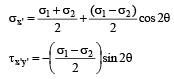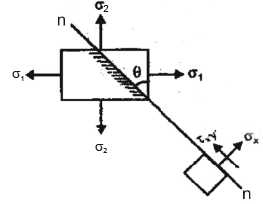Case-2 : If σx and σy are normal stresses and txy is shear stress acting on the mutually perpendicular planes then the normal and shear stresses on any plane n-n inclined at an angle θ with the plane of σx are given by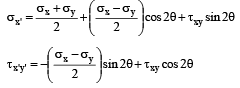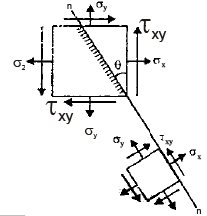Special case-1 : If θ becomes such that ζx'y' on this plane becomes zero then this plane will be known as principal plane and the angle of principal plane is given by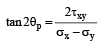The magnitude of principal stresses σ1 and σ2 are given by

σor σ2 = (σxy)/2 ± √[(σxy/2)22]

Special case-2 : The plane of maximum shear stress lies at 45° to the plane of principal stress and magnitude of ζmax is given by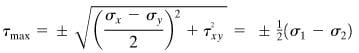Note that planes of ζmax carry equal and alike normal stresses. The normal stress on plane of  ζmax is given by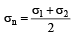Therefore resultant stress on the plane of Тmax is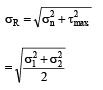The angle of obliquity of σr with the direction of σn is given by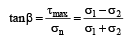Special case-3 : In case of pure shear element, the principal stresses act at 45° to the plane of pure shear stress.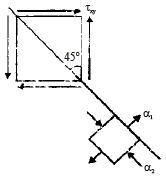σ1 = +  ζxy
σ= – ζxy ·

• Properties of Mohr’s Circle for Stress :
•  Mohr’s circle is the locus on normal and shear stresses on an element with the changing angle of plane in 2 dimensional case.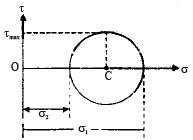The radius of Mohr’s circle is equal to maximum shear stress.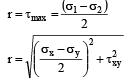• The centre of circle always lies on s axis and its co-ordinates are (σn, 0)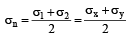Note : Sum of normal stresses on two mutually perpendicular planes remain constant i.e.σ1 + σ2 = σ+ σy = constant

• In case of pure shear element σ1 = + ζ and σ2 = – ζ therefore centre of the circle coincides with the origin,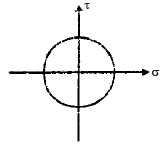• In case of an element inside the static fluid, ζ = 0 because principal stresses are equal and alike therefor Mohr’s circle reduces into a point

COMBINED BENDING & TORSION
Let a shaft of diameter ‘d’ be subjected to bending moment ‘M’ and a twisting moment ‘T’ at a section. At any point in the section at radius ‘r’ and at a distance y from the neutral axis, the bending stress is given by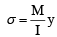and shear stress is given by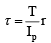Where I = Moment of inertia about its NA and Ip = Polar moment of Inertia.

•  The location of the principal planes through the point is given by tan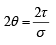•  The principal stress es are given by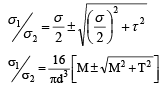• The maximum shear stress is given by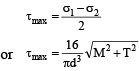• The position of principal planes is given by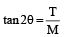EQUIVALENT BENDING MOMENT & EQUIVALENT TORQUE

• Let ‘Me’ be the equivalent bending moment which acts alone producing the maximum tensi l e stress equal to σ1, as produced by M and T.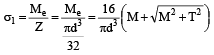Therefore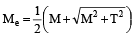• Let ‘Te’ be the equivalent torque, which acts alone producing the same maximum shearing stress tmax as produced by M and T.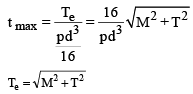ANALYSIS OF PRINCIPAL STRAINS

•  Case-1 : If ε1 and ε2 are principal strains in two mutual perpendicular directions in plane stress problem then principal stresses are given by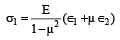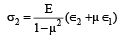• If ε1 and ε2 are principal strains in x and y directions respectively, then normal and shear strain in any other direction x' are given by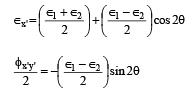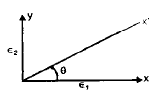• If εx, εy and φxy are normal and shear strain in x – y plane the normal and shear strain in x' – y' plane are given by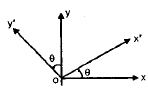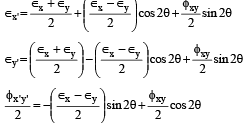Special case : If φx'y' = 0 then magnitude of principal strains and their plane are given by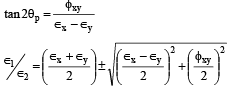• · Properties of Mohr’s circle for strain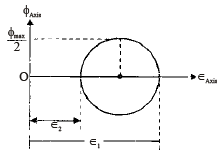The radius of Mohr’s circle is half of maximum shear strain i.e.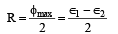Therefore Diameter of Mohr’s circle,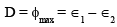σ = (P/A)
When load is applied suddenly, then the normal stress ‘σ’ due to load P is given by
σ = (2P/A)

Hence, maximum stress intensity due to suddenly applied load is twice the stress intensity produced by the load of the same magnitude applied gradually.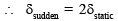THEORIES OF ELASTIC FAILURE

• Function of theories of elastic failure is to predict the behaviour of materials in simple tensile test when elastic failure will occur under any condition of applied stress.
• Maximum principal stress theory (Rankine) This assumes that max. principal stress in the complex system reaches the elastic limit stress in simple tension and failure occurs when σ1 = σy for tension Failure can occur in compression when least principal stress (σ3) reaches the elastic limit stress in compression i.e. σ3 = σy  for compression
• It is well suited for brittle materials.

Failure envelope occurs when
(a) σ1 or σ2 = σyt  or  σyc
(ii) σ3 = 0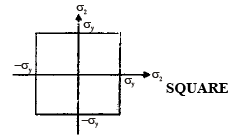• Max shear stress theory (Guest - Tresca) This assumes that max shear stress in the  complex stress system becomes equal to that at the yield point in simple tensile test.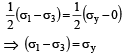• This theory holds good for ductile materials. For like stresses in Ist and IIIrd quadrant σ1 = σy or σ2 = σy

• For unlike stresses in IInd or IVth quadrant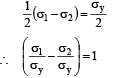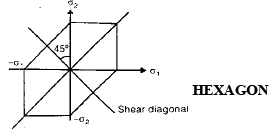Note : Aluminium alloys & certain steels are not governed by the Guest theory.

• Max principal strain theory (Saint Venant) This assumes that failure occurs when max. strain in the complex stress system equals that at the yield point in the tensile test (σ1 – µσ2 – µσ3) = σy
Failure should occur at higher load because the Poisson's ratio reduces the effect in perpendicular directions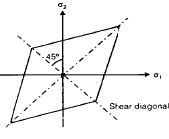RHOMBUS

•  Maximum strain energy theory (Haigue)or Total strain Energy Theory : -This assumes that failure occur when total strain energy in the complex system is equal to that at the yield point in tensile test.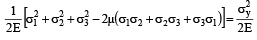It is fairly good for ductile materials.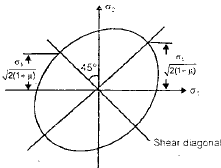ELLIPSE

•  Maximum shear strain energy theory or distortion energy theory (Mises-Henky Theory).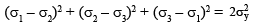The properties are similar in tension and compression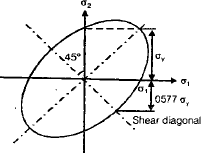ELLIPSE•  Failure of most ductile materials is governed by the distortion energy criterion or Von mises theory.
σ1 = σ2 = σ3; cylinder
σ= σ2 and σ3 = 0; ellipse ·

• Factor of safety =  Tensile strength / Allowable working stress
The document Principal Stress-Strain & Theories of Failure Notes | Study Civil Engineering SSC JE (Technical) - Civil Engineering (CE) is a part of the Civil Engineering (CE) Course Civil Engineering SSC JE (Technical).
All you need of Civil Engineering (CE) at this link: Civil Engineering (CE)Use Code STAYHOME200 and get INR 200 additional OFF

## Civil Engineering SSC JE (Technical)

2 videos|111 docs|50 tests

Track your progress, build streaks, highlight & save important lessons and more!

,

,

,

,

,

,

,

,

,

,

,

,

,

,

,

,

,

,

,

,

,

;